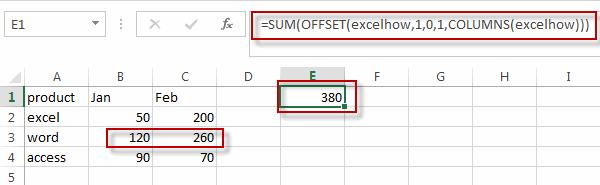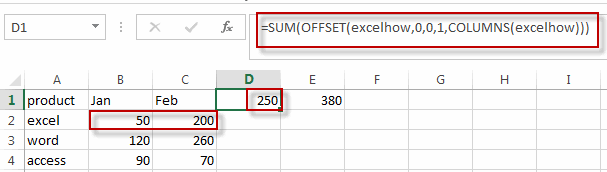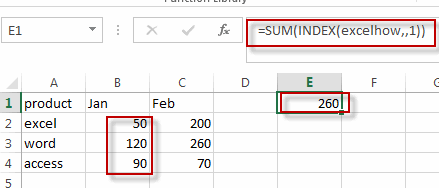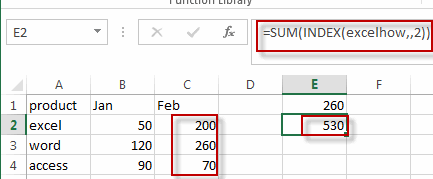# Sum Specific Row or Column in Named Range

This post will guide you how to sum a specific Row or Column in a named range in excel. Normally, you can easily sum a certain row or column in a worksheet in excel. But if you just want to sum a particular column or row in a named range, how to achieve it in excel.

## Sum Specific Row in Named Range

Assuming that you have a name range “excelhow”, if you want to sum a specific row (row 2) in named range, you need to create a formula based on the SUM function and the OFFSET function and the COLUMNS function to achieve the reuslt. Just like this:

`=SUM(OFFSET(excelhow,1,0,1,COLUMNS(excelhow)))`Type this formula into a blank cell and then press enter key in your keyboard, you will see that the second row in the named range has been summed.

If you want to sum the first row in the named range “excelhow”, you just need to change the first “1” number as “0”. Just like the below formula:

`=SUM(OFFSET(excelhow,0,0,1,COLUMNS(excelhow)))`## Sum Specific Column in Named Range

If you want to sum a specific column (column1) in the named range “excelhow”, you need to create a formula with the SUM function in combination with the INDEX function. Just like this:

`=SUM(INDEX(excelhow,,1))`If you want to sum the second column in the named range “excelhow”, you just need to change the number “1” in this formula as “2”.

`=SUM(INDEX(excelhow,,2))`### Related Functions

• Excel SUM function
The Excel SUM function will adds all numbers in a range of cells and returns the sum of these values. You can add individual values, cell references or ranges in excel.The syntax of the SUM function is as below:= SUM(number1,[number2],…)…
• Excel INDEX function
The Excel INDEX function returns a value from a table based on the index (row number and column number)The INDEX function is a build-in function in Microsoft Excel and it is categorized as a Lookup and Reference Function.The syntax of the INDEX function is as below:= INDEX (array, row_num,[column_num])…
• Excel Columns function
The Excel COLUMNS function returns the number of columns in an Array or a reference.The syntax of the COLUMNS function is as below:=COLUMNS (array)….

Related Posts

6 Best Free Wedding Budget Templates

This post will show you some free wedding budget spreadsheet templates for both google sheets and Microsoft Excel Spreadsheet, as well as some explanations of the features or important function points of these templates. You can then easily make modifications ...

Calculate Cumulative Totals with Excel SUM Function

Today, through a simple example, we will show you how to use one of the most common-used Mathematical functions in excel, the SUM function, to add up the sum. In our daily life, we keep an account of what we ...

Check Dates in chronological order

Assume you have a date list that has different date formats, as seen in the accompanying picture. In this instance, Excel's Sort function will fail to sort them appropriately. However, you may convert all various date formats to a particular ...

How to Use 3D SUM Multiple Worksheets

To sum a range of numbers is straightforward for most Excel users, but do you know how to establish a 3D reference to total the same range of numerous sheets. In this post, I will present the steps for this ...

If Cell Begins with One of Three Supplied Characters

If you want to test values to see if they begin with some given specific characters like “x”, ”y”, or “z”, you can create a formula with COUNTIF and SUM functions to return results. EXAMPLE You can see “TRUE” or ...

Average per Week by Formula in Excel

We usually apply AVERAGE function or relevant functions to return average directly in Excel worksheet. But in some situations, only applying average relevant functions cannot figure out our problem. Sometimes we can create a formula with functions and mathematical operation ...

How to Sum if Contains an Asterisk

To add numbers together we need to apply SUM function. And if we want to add numbers based on some conditions, we can add criteria with the help of SUMIFS function, SUMIFS can filter data with multiple criteria effectively. If ...

How to Sum in Vertical Range

If we want to add numbers based on some conditions in Excel worksheet, we can add criteria with the help of SUMIFS function, SUMIFS can filter data with multiple criteria effectively. In this article, we will introduce you the method ...

How to Sum in Horizontal Range

To add numbers together we need to apply SUM function. But if we want to add numbers based on some conditions, we can add criteria with the help of SUMIFS function, SUMIFS can filter data with multiple criteria effectively. In ...

How to Sum with Criteria and Or Logic in Excel

To add numbers together we need to apply SUM function. And if we want to add numbers based on some conditions, we can add criteria with the help of SUMIFS function, SUMIFS can filter data with multiple criteria effectively. If ...

Sidebar